# Gromov-Lawson conjecture

(diff) ← Older revision | Latest revision (diff) | Newer revision → (diff)

If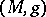is a metric of positive scalar curvature (cf. also Metric) on a compact spin manifold (cf. also Spinor structure), results of A. Lichnerowicz [a4] show that there are no harmonic spinors; consequently, the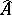-genus ofvanishes. M. Gromov and H.B. Lawson [a2], [a3] showed that if a manifoldcan be obtained from a manifold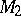which admits a metric of positive scalar curvature, by surgeries in codimension at least, then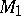admits a metric of positive scalar curvature. They wondered if this might be the only obstruction to the existence of a metric of positive scalar curvature in the spinor context if the dimensionwas at least. (This restriction is necessary to ensure that certain surgery arguments work.) S. Stolz [a9] showed this was the case in the simply-connected setting: ifis a simply-connected spin manifold of dimension at least(cf. also Simply-connected domain), thenadmits a metric of positive scalar curvature if and only if the-genus ofvanishes. This invariant takes values in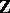if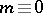modulo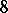, in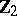if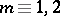modulo, in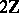if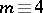modulo, and vanishes if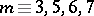modulo.

The situation is more complicated in the presence of a fundamental group. Let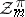be the Grothendieck group of finitely generated-graded modules over the Clifford algebrawhich have aaction commuting with the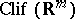action. The inclusionofin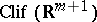induces a dual pull-back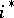fromto. The real-theory groups of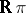are given by:J. Rosenberg [a6] defined a-theory-valued invarianttaking values in this group which generalizes the-genus. It was conjectured that this might provide a complete description of the obstruction to the existence of a metric of positive scalar curvature; this refined conjecture became known as the Gromov–Lawson–Rosenberg conjecture.

The conjecture was established for spherical space form groups [a1] and for finite Abelian groups of rank at mostand odd order [a8]. It has also been established for a (short) list of infinite groups, including free groups, free Abelian groups, and fundamental groups of orientable surfaces [a5]. S. Schick [a7] has shown that the conjecture, in the form due to J. Rosenberg, is false by exhibiting a compact spin manifold with fundamental groupwhich does not admit a metric of positive scalar curvature but for which theinvariant vanishes. It is not known (1998) if the conjecture holds for finite fundamental groups.

How to Cite This Entry:
Gromov-Lawson conjecture. Encyclopedia of Mathematics. URL: http://encyclopediaofmath.org/index.php?title=Gromov-Lawson_conjecture&oldid=11970
This article was adapted from an original article by P.B. Gilkey (originator), which appeared in Encyclopedia of Mathematics - ISBN 1402006098. See original article